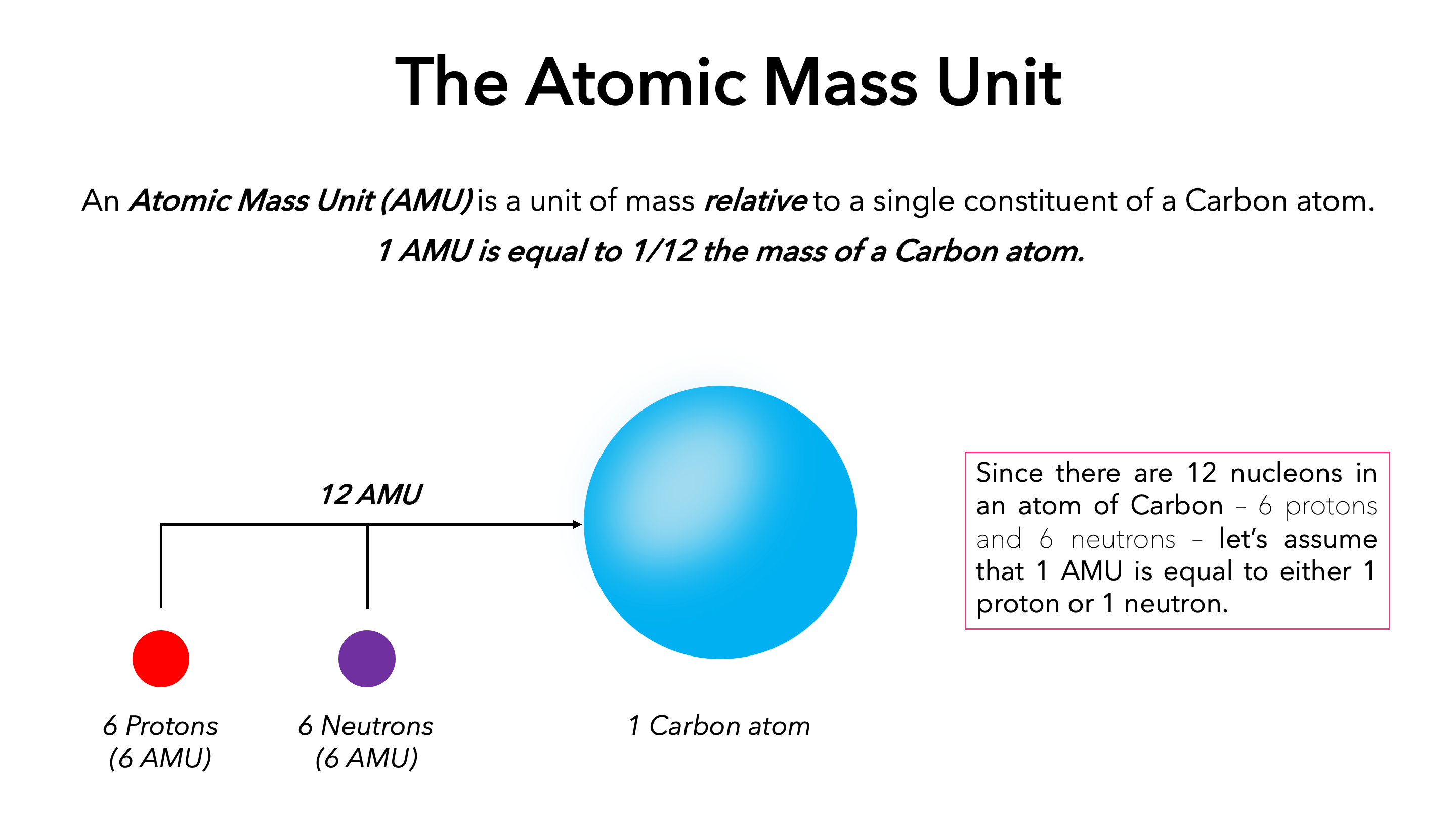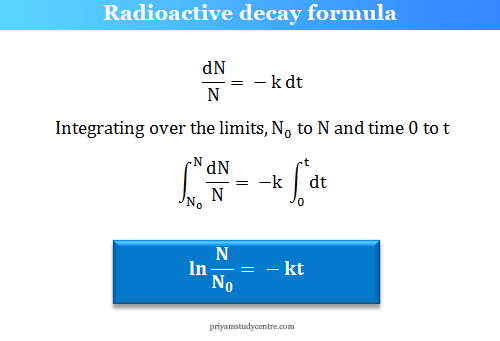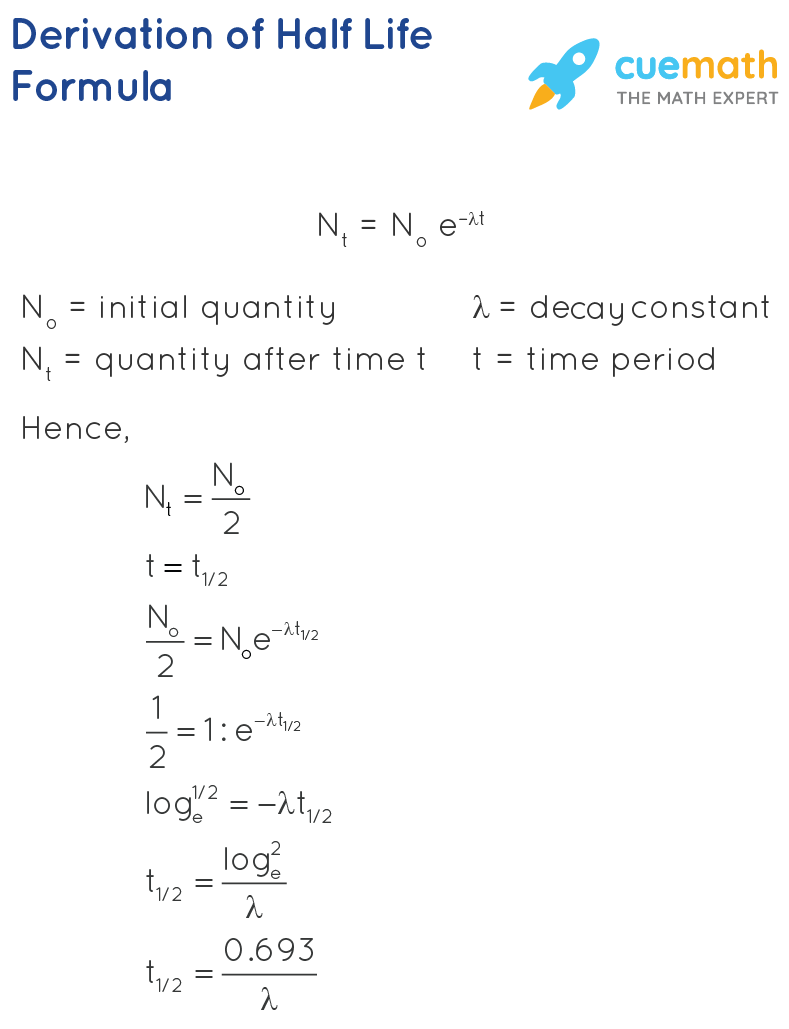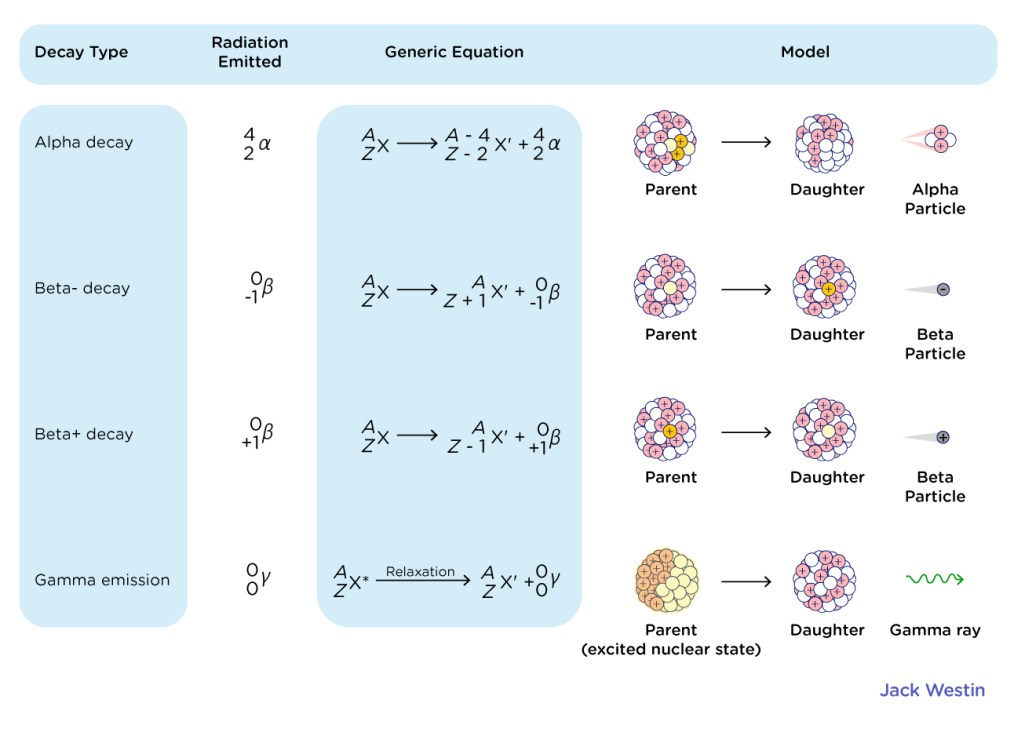# CBSE Class 12 Physics Chapter 13 Revision Notes

Chapter 13: Nuclei Revision Notes

• The positive charge and mass of each atom are densely concentrated in the nucleus, which forms the atom’s center. In the nucleus, more than 99.9% of the atom’s mass is concentrated.
• Atomic Mass Unit (amu): The atomic mass unit is the unit used to express atomic masses. 1/12th of the mass of a carbon atom is defined as an atomic mass unit (C12).Source

## Composition of Nucleus

The following terms and symbols can be used to describe the nucleus’s composition.

• Atomic Number Z: An element’s atomic number is the number of protons contained within the nucleus of one of its atoms.

Number of protons = Number of electrons = Atomic number

• The total number of protons and neutrons inside the atomic nucleus of an element is known as its mass number.

A=Z.+ N = Number of protons + Number of neutrons = Number of electrons + Number of neutrons

• It is the spontaneous disintegration of an atom’s nucleus, accompanied by the emission of one or more radiations such as α-particle, β-particle or γ-rays.
• Radioactive Decay: It is a nuclear transformation process in which the nucleus of the atom emits radioactive rays. Any physical or chemical process cannot speed up or slow down this process.
• Radioactive Decay Law: The rate of decay of radioactive atoms is proportional to the number of atoms present at any given time, according to this law.Source

• Half-Life: The half-life of a radioactive element is defined as the time taken for half of the atoms in a sample of the element to decay.Source

• Average Life/Mean Life: The average life of a radioactive element is calculated by dividing the total lifetime of all the radioactive element’s atoms by the total number of atoms present in the element’s sample at the start.
• Decay Constant: The reciprocal of the time it takes for the number of atoms in a radioactive substance to reduce to 36.8% of their initial number is the radioactive decay constant.Source

• The laws of radioactive displacement are also known as Fajan’s and Soddy’s laws. This law specifies which chemical elements and isotopes are produced during a specific type of radioactive decay.
• α- Decay: In α-decay, the product nucleus has a mass number four times that of the decaying nucleus, while the atomic number decreases by two.
• β-Decay: The mass number of the product nucleus remains constant during decay, but the atomic number increases or decreases by one.
• An electron and an antineutrino are created and emitted from the nucleus in beta-minus decay (β).
• A positron and a neutrino are created and emitted from the nucleus in beta-plus decay (+) via the reaction given below:
• γ-Decay: When an or -decay results in an excited daughter nucleus, a -ray is emitted. The atom then returns to its ground state via a single photon transition or a series of photon transitions.
• The Becquerel is the SI unit of radioactivity (Bq).
• 1 Becquerel(Bq) = 1 second of disintegration
]]>